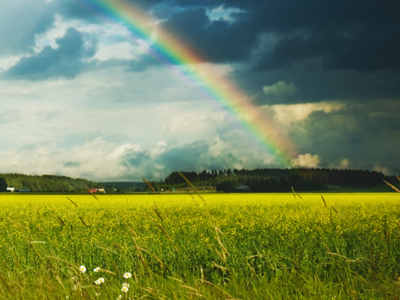The name of a part of the circumference of a circle is arc, such as the arc of a rainbow.

# Shapes 4

This Math quiz is called 'Shapes 4' and it has been written by teachers to help you if you are studying the subject at middle school. Playing educational quizzes is a fabulous way to learn if you are in the 6th, 7th or 8th grade - aged 11 to 14.

It costs only \$12.50 per month to play this quiz and over 3,500 others that help you with your school work. You can subscribe on the page at Join Us

Shapes with equal angles at teach of their corners, like squares or pentagons, are called regular shapes. But what do we call a shape with different angles, like a kite, a rhombus or a parallelogram? We call it an irregular shape. Irregular shapes have some odd names, like trapezium or even diamond!Cubes and pyramids are 3D shapes. Making three-dimensional shapes with card can be great fun and will give you an idea of how many faces a certain shape has.

Do you know the difference between a rhombus and a parallelogram? Test your knowledge of trapeziums, dodecahedrons and some other shapes in this quiz.

1.
What are perpendicular lines?
Perpendicular lines are lines that are getting closer and closer to each other
Perpendicular lines are lines that are always the same distance apart
Perpendicular lines are lines that are at right angles to each other
Perpendicular lines are lines that are short
The corners of rectangles are formed by perpendicular lines
2.
What is the name of a 12 sided flat shape?
Dodecagon
Nonagon
Heptagon
Decagon
'Do' means 2 and 'deca' means 10 so 'dodeca' means 12
3.
What is the name of two lines that are always the same distance apart?
Parallel
Perpendicular
Convergent
They never cross each other
4.
What do two concentric circles share?
Center point
Equal circumference
Equal diameter
Ripples on the surface of a pond are concentric circles
5.
Which quadrilateral has no parallel sides?
Square
Kite
Rhombus
Rectangle
A kite has 2 pairs of equal length sides but none of them are parallel to another
6.
What is the name of a part of the circumference of a circle?
Arc
Center
Diameter
Rainbows are arcs
7.
A rhombus looks like which other shape?
A kite
A square
A triangle
A diamond
A rhombus is a quadrilateral with 4 equal length sides, like a square that has been squashed!
8.
Which of these shapes is not a parallelogram?
Square
Rectangle
Rhombus
Trapezium
A parallelogram has all opposite sides parallel and equal in length
9.
What is the name given to the distance around the edge of circle?
Diameter
Tangent
Circumference
'Circum' means going around and is related to the word 'circle'
10.
How many faces does a dodecahedron have?
8
10
12
14
A regular dodecahedron has pentagon shaped faces
Author:  Amanda Swift